Mobile QR Code1. (Department of Electronic Engineering, Kumoh National Institute of Technology, Gumi, Gyungbuk, Korea)

Successive approximation register, noise shaping, analog-to-digital converter, differential capacitor, voltage gain calibration

## I. INTRODUCTION

Smart devices require small area and low-power analog-to-digital converters (ADCs) to acquire information such as temperature, pressure, and Earth’s magnetic field. In addition, the resolution of ADCs required in sensor interfaces continues to increase. Delta-sigma ADCs have been widely used for high-resolution sensor interfaces. However, since they generally use analog integrators with operational amplifiers, the power consumption and area are relatively large [1-3]. Furthermore, the high oversampling ratio used in the delta-sigma modulator increases the frequency of the sampling clock and the complexity of the decimation filter.

On the other hand, a successive approximation register (SAR) ADC has advantages of low power consumption and small area. However, it has difficulty in increasing the resolution due to the area and mismatch of the digital-to-analog converter (DAC) [4-6]. As an alternative to the limitation of improving the resolution of SAR ADCs, SAR ADCs using noise shaping (NS) are being studied [7-9]. Passive integrators can be used for noise shaping without increasing power consumption [7,8]. In this case, the capacitor for the passive integrator increases the area of the NS SAR ADC. In addition, the comparator for the operation of the NS SAR ADC is designed to receive and compare multiple input signals rather than a single input. For the stability and performance of the NS SAR ADC, it is required that the comparator has an optimal voltage gain for the output of the passive integrator.

In this paper, a NS SAR ADC that increases resolution by applying second-order noise shaping in a 10-bit SAR ADC is proposed. In particular, the use of a capacitor-resistor hybrid DAC (C-R DAC) for operation of SAR ADC and two differential capacitors for second-order noise shaping is proposed to minimize the area of the NS SAR ADC [10-12]. Furthermore, voltage gain calibration for a three-input comparator is proposed for stable noise shaping and evaluated from the measurement results of the NS SAR ADC. Section II presents the behavioral model and simulation results for the design of the second-order NS SAR ADC. Section III explains the operation including the block and timing diagrams of the proposed NS SAR ADC. It describes a design technique to minimize the area of the NS SAR ADC and a three-input comparator with voltage gain calibration for second noise shaping. Section IV presents the implementation and measurement results of the NS SAR ADC. Finally, Section V provides the conclusion of this paper.

## II. BEHAVIORAL MODEL OF SECOND-ORDER NOISE SHAPING SAR ADC

Fig. 1 shows the conceptual block diagram of the proposed second-order NS SAR ADC. The proposed second-order NS SAR ADC basically has an architecture in which a second-order integrator is added to a 10-bit SAR ADC. The SAR ADC is simply represent by using a 1-bit quantizer, a SAR logic, and a DAC, and outputs a 10-bit binary digital code as the result of the NS SAR ADC. H(z) is a second-order integrator for noise shaping. The second-order NS SAR ADC is different from a general SAR ADC in the two way: 1) H(z) outputs V$_{\mathrm{INT1}}$(z) and V$_{\mathrm{INT2}}$ by integrating V$_{\mathrm{RES}}$(z), which is the residue voltage after quantization of the SAR ADC. 2) V$_{\mathrm{RES}}$(z) is summed with V$_{\mathrm{INT1}}$(z) and V$_{\mathrm{INT2}}$(z), and the summed analog signal, V$_{\mathrm{QIN}}$(z), is converted to a 10-bit digital code by the operation of the SAR ADC.

##### Fig. 1. Conceptual block diagram of NS SAR ADC: (a) NS SAR ADC; (b) second-order integrator.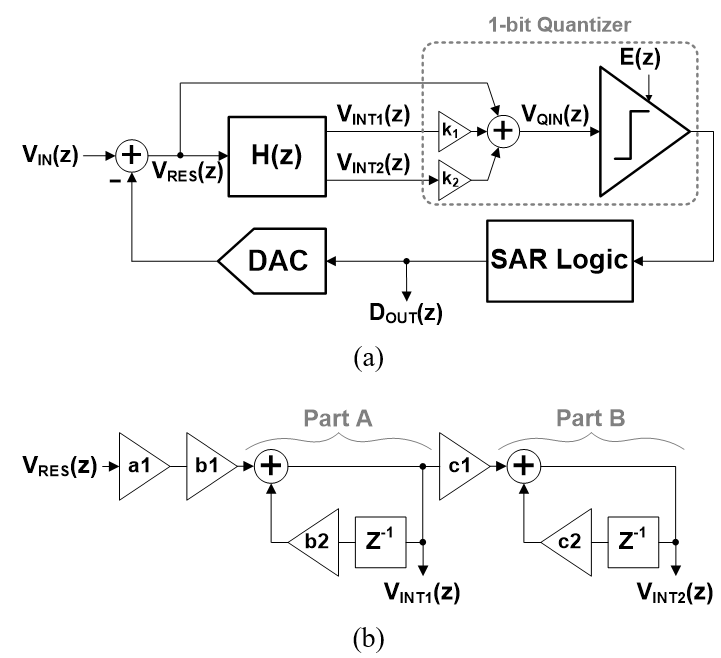The behavioral model of the second-order integrator, H(z) is shown in Fig. 1(b). Part A and Part B are blocks for the first and second integration, respectively. In this work, the second-order integrator is implemented by using passive filters consisting only of capacitors and switches without amplifiers. a1 is the coefficients for voltage reduction due to charge sharing between the C-R DAC of the SAR ADC and the sampling capacitor of the integrator. In addition, b1 and b2, and c1 and c2 are coefficients for voltage reduction due to charge sharing between the sampling capacitor of the integrator and the capacitors of the first and second integrators, respectively. The outputs of the two integrators, V$_{\mathrm{INT1}}$ and V$_{\mathrm{INT2}}$, are defined as Eqs. (1) and (2), respectively, when a1, b1, b2, c1, and c2 are 3/4, 1/4, 3/4, 1/4, and 3/4, respectively.

##### (1)
V INT 1 Z = 0 . 25 0 . 75 1 0 . 75 Z 1 V RES ( Z )
##### (2)
V INT 2 Z = 0 . 25 2 0 . 75 1 0 . 75 Z 1 2 V RES ( Z )

The output of the proposed NS SAR ADC, D$_{\mathrm{OUT}}$(z), is derived as Eq. (3) by the behavioral block diagram shown in Fig. 1(a), where the voltage gains of the comparator for V$_{\mathrm{INT1}}$ and V$_{\mathrm{INT2}}$, k$_{1}$ and k$_{2}$, are set to 4 and 16 for the stable NTF, respectively . Eq. (3) is simply expressed as Eq. (4) by using Eqs. (1) and (2) for the V$_{\mathrm{INT1}}$ and V$_{\mathrm{INT2}}$. Therefore, the analog input signal V$_{\mathrm{IN}}$(z) is directly transferred to the output of the NS SAR ADC, and the quantization noise E(z) is shaped by the noise transfer function (NTF) shown in Eq. (5).

##### (3)
D OUT Z = V IN Z + 1 1 + 4 V INT 1 Z + 16 V INT 2 Z Z 1 E ( Z )
##### (4)
D OUT Z = V IN Z + 1 0 . 75 Z 1 2 E ( Z )
##### (5)
NTF = 1 0 . 75 Z 1 2

Fig. 2 shows the behavioral simulation results of the NTF. The NTF of the designed NS SAR ADC has the characteristics of a high-pass filter in which the voltage gain decreases in the low-frequency region and increases as the frequency increases. Through such shaping of quantization noise, it is possible to increase the signal-to-noise ratio (SNR) of data conversion for an analog input signal with low frequency.

##### Fig. 2. Behavioral simulation result of NTF.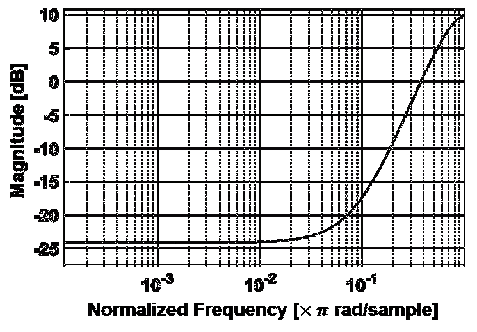Fig. 3(a) shows the behavioral model of the proposed second-order NS SAR ADC based on a 10-bit SAR ADC using the MATLAB Simulink. It consists of a 1-bit quantizer, a SAR logic with DAC, and a second-order integrator are implemented by components supporting the MATLAB Simulink. This behavioral model does not include non-ideal components of the NS SAR ADC. The analog input of the second-order NS SAR ADC is sampled through the process A. The process B shows that the reference voltage for the operation of the 10-bit SAR ADC, which is generated by the SAR logic and the DAC with a 9-bit resolution, is supplied to the 1-bit quantizer. In the process C, the residue voltage, V$_{\mathrm{RES}}$, is generated after the conversion of the 10-bit SAR ADC, and is sampled for the integration operation. It is determined by the difference between the sampled input voltage and the output voltage of the 10-bit DAC including the information of the least significant bit of the 10-bit SAR ADC for the noise shaping operation, although the 10-bit SAR ADC requires the reference voltages generated by the 9-bit DAC, as shown in Fig. 3(b). The second-order integrator supplies the integrated residue voltage to the 1-bit quantizer in the process D. The 1-bit quantizer compares the sum of the sampled input and the two integrated residue voltages against a reference that changes sequentially 9 times for operation of the 10-bit SAR ADC. The 1-bit quantizer operates in synchronization with the signal of COMP_CLK.

##### Fig. 3. (a) Behavioral model of proposed NS SAR ADC; (b) residue voltage for noise shaping.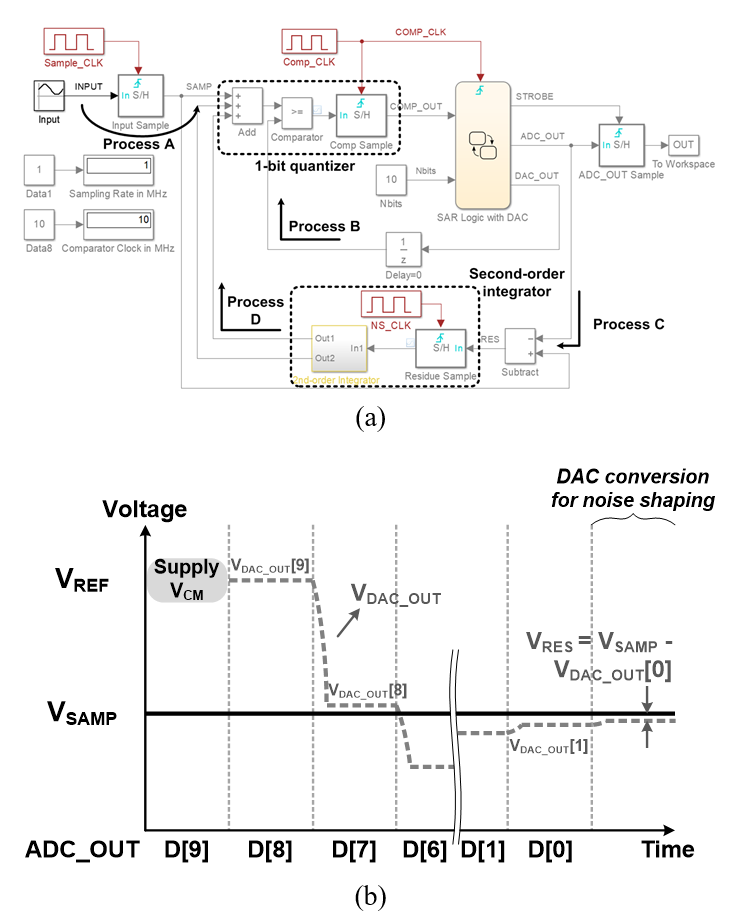Fig. 4 shows the behavioral model of the SAR logic with DAC. The SAR logic with DAC is implemented by using a Chart in the Stateflow library of the MATLAB Simulink which can implement a control logic with finite state machine. Bit is a variable representing the number of SAR conversions of 10 times, and DAC_OUT is the output of the 9-bit DAC. The value of Bit is from 1 to 10 for 10-bit SAR operation, and its initial value is 1. Since the analog input range of the proposed second-order NS SAR ADC is 0 V to 1.8 V, the initial value of DAC_OUT is set to 0.9 V, which is the center voltage. The SAR logic with DAC is synchronized with the signal of COMP_CLK and operates according to the result of the 1-bit quantizer, COMP_OUT. If the value of Bit is less than 10, the signal STROBE indicating the completion of the SAR operation is maintained low, and whenever the signal COMP_CLK goes high, the value of Bit is increased by 1. In this process, the 9-bit DAC outputs the reference voltage as the value of DAC_OUT by the successive approximation algorithm. When the value of Bit is 10, the signal STROBE goes high, and DAC_OUT is sampled as the residue value for integration of noise shaping by the signal STROBE. After that, Bit and DAC_OUT are initialized.

##### Fig. 4. Behavioral model of SAR logic with DAC.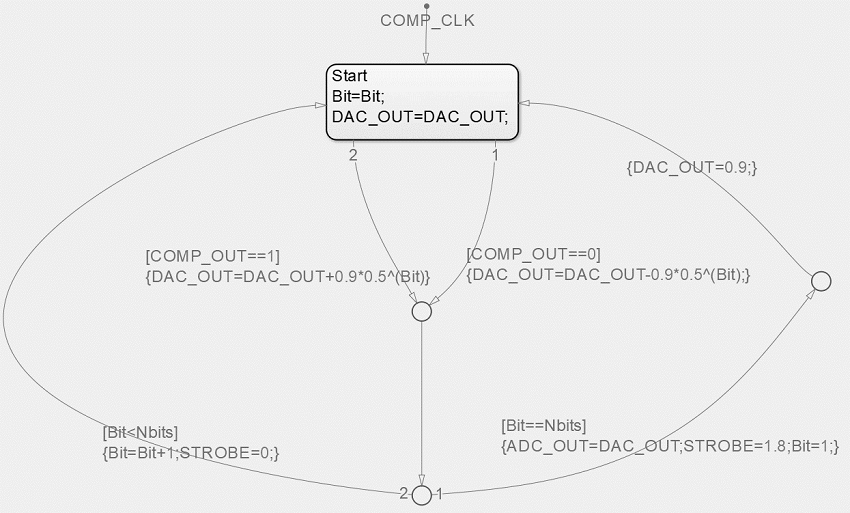##### Fig. 5. Behavioral simulation results: (a) 10-bit SAR ADC; (b) proposed second-order NS SAR ADC.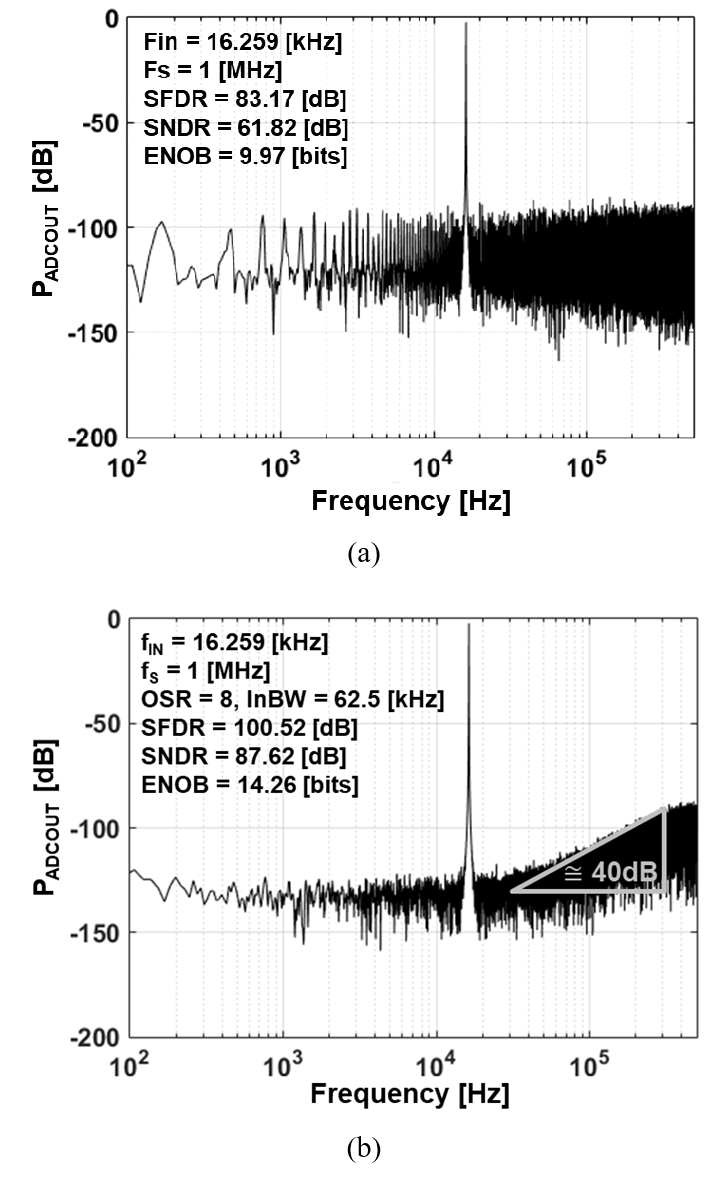## III. DESIGN OF SECOND-ORDER NOISE SHAPING SAR ADC

### 1. Architecture and Operation of NS SAR ADC

Fig. 6 shows the block and timing diagrams of the proposed second-order NS SAR ADC. The second-order NS SAR ADC consists of a 10-bit digital-to-analog-converter (DAC), integration capacitors for noise shaping, a three-input comparator, and a SAR logic including gain calibration, as shown in Fig. 6(a). Its OSR and input bandwidth are 8 and 62.5 kHz. The three-input comparator generates the signal COMP_OUT by differentially comparing the sum of the three results, the result of the 10-bit DAC and the outputs of the first and second integrators. The 10-bit DAC has a C-R DAC architecture, which consists of an 8-bit capacitor DAC and a 2-bit resistor DAC. The C-R DAC can reduce the chip area compared with a DAC using only capacitors. In addition, the use of the C-R DAC can decrease the capacitor values used in the passive integrator for noise shaping because the capacitor value used in the passive integrator is determined proportional to the total capacitor value of the C-R DAC generating the residue voltage. The noise shaping is performed by using passive integrators, which consists of two C$_{\mathrm{RES}}$s, one C$_{\mathrm{INT1}}$, and one C$_{\mathrm{INT2}}$. C$_{\mathrm{INT1}}$ and C$_{\mathrm{INT2}}$ are used as differential capacitors to reduce the increase in chip area due to noise shaping using a passive integrator. The two C$_{\mathrm{RES}}$s are used to perform the sampling operation for the residue voltage of the 10-bit DAC. The first and second integrations are performed by C$_{\mathrm{INT1}}$ and C$_{\mathrm{INT2}}$, respectively, sequentially sharing charges with C$_{\mathrm{RES}}$.

##### Fig. 6. Proposed NS SAR ADC: (a) block diagram; (b) timing diagram.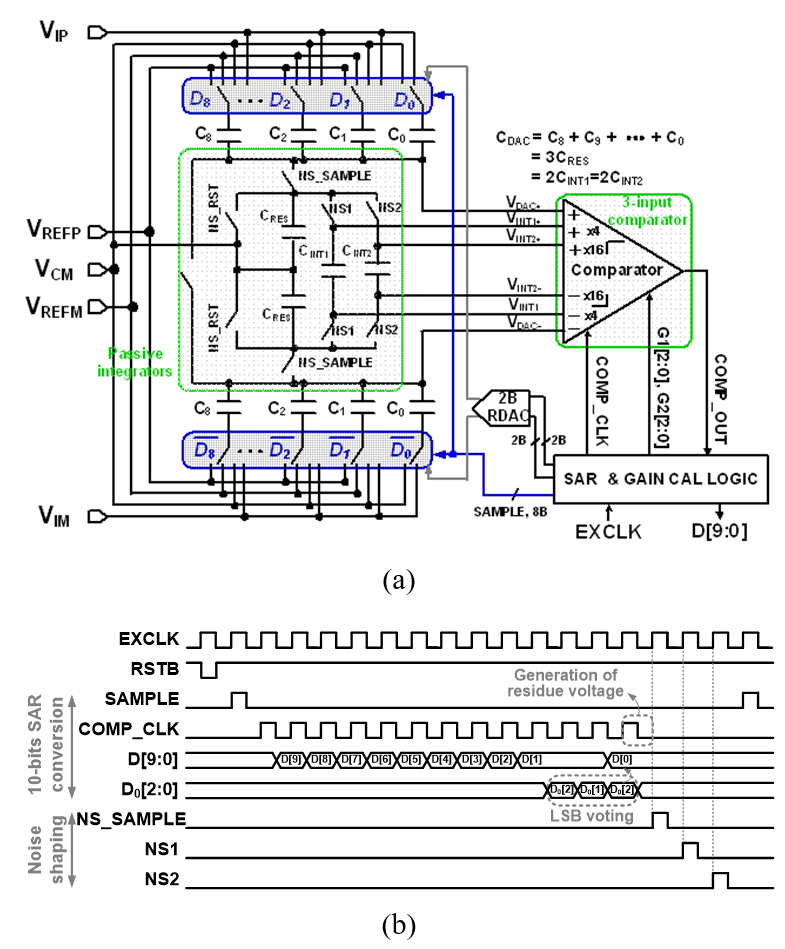The proposed second-order NS SAR ADC operates in synchronization with the external clock EXCLK with a frequency of 17 MHz. It samples the input analog signal during the high region of the first cycle of EXCLK after the signal RSTB goes high, as shown in Fig. 6(b). After that, the SAR data conversion proceeds in synchronization with the rising edge of COMP_CLK, which is the comparator operation clock. The output of the second-order NS SAR ADC, D[9:0], is sequentially output in synchronization with the falling edge of COMP_CLK. The least significant bit, D, is decided by voting from D$_{0}$, D$_{0}$, and D$_{0}$ as a results of three comparisons for the same output of the 10-bit DAC to remove the dynamic noise of the comparator. For noise shaping, the residue voltage generated in the 10-bit DAC by the 10-bit SAR conversion is sampled in C$_{\mathrm{RES}}$ by the signal NS_SAMPLE. The first integrator generates an output by sharing charges between C$_{\mathrm{RES}}$ and C$_{\mathrm{INT1}}$ when the signal NS1 is high. After the first integration, the output of the second integrator is generated as C$_{\mathrm{RES}}$ again shares charges with C$_{\mathrm{INT2}}$, as shown in Fig. 6(a).

Table 1shows the total capacitors used for the implementation of a 10-bit SAR ADC and noise shaping, and explains the size of the capacitor reduced by the C-R DAC and differential capacitor used in this work. C is the value of the unit capacitor used in the NS SAR ADC. For operation of 10-bit SAR analog-to-digital conversion ADC and generation of residue voltage, a differential capacitor DAC using a V$_{\mathrm{CM}}$-based switching scheme is implemented by using 2048${\cdot}$C. In addition, C$_{\mathrm{RES}}$, C$_{\mathrm{INT1}}$, and C$_{\mathrm{INT2}}$ are determined to be 1/3${\cdot}$C$_{\mathrm{DAC}}$, 1${\cdot}$C$_{\mathrm{DAC}}$, and 1${\cdot}$C$_{\mathrm{DAC}}$, respectively, to implement the coefficients $\textit{a1, b1, b2}$, $\textit{c1}$, and $\textit{c2}$ of the passive integrators shown in Fig. 1(b) as 3/4, 1/4, 3/4, 1/4, and 3/4. Thus, the design method reported in the prior literature  requires a total capacitor of 6826.6${\cdot}$C to implement the second-order NS SAR ADC while the SAR ADC has a resolution of 10 bits. A 10-bit C-R DAC with a 2-bit resistor DAC can reduce the capacitor size to 1/4 that of a 10-bit CDAC. Furthermore, the use of differential capacitors C$_{\mathrm{INT1}}$ and C$_{\mathrm{INT2}}$ for integration halves the number and value of capacitors, so that C$_{\mathrm{INT1}}$ and C$_{\mathrm{INT2}}$ can be determined to be 1/4 of the size of the conventional integrator capacitors. The proposed second-order NS SAR ADC uses 938.6${\cdot}$C by using the C-R DAC and the two differential capacitors. Therefore, it has a capacitor area reduced by 86.25% compared to the conventional NS SAR ADC.

##### Table 1. Size comparison of capacitors used in second-order NS SAR ADC.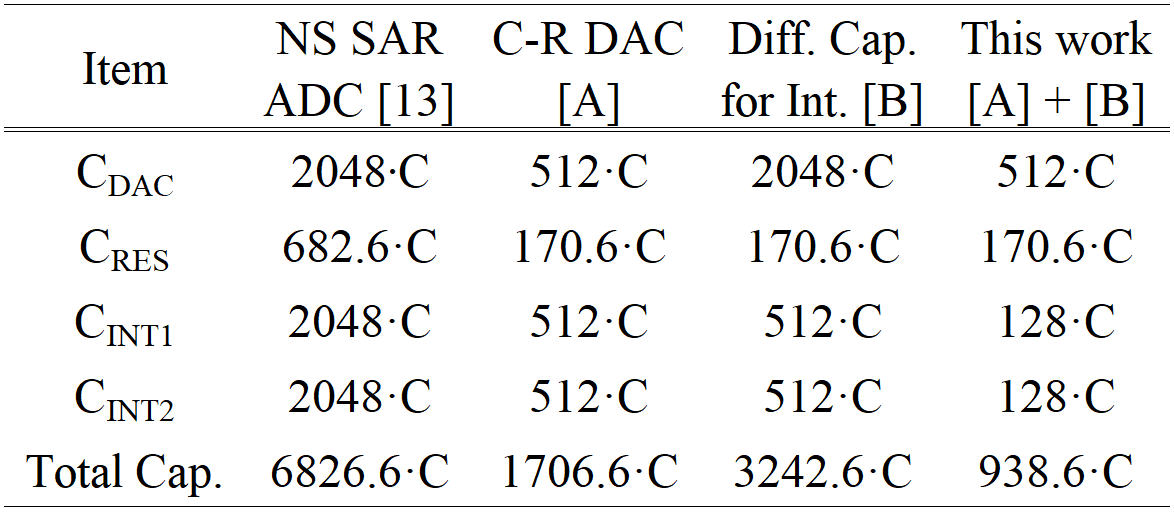### 2. Second-order Integrator

Fig. 7 shows the circuit and operation of the second-order integrator used in the proposed second-order NS SAR ADC. First, after the 10-bit SAR conversion is completed, the generated residual voltage is stored in the C-R DAC, as shown in Fig. 7(a), where C$_{\mathrm{DACP/M}}$ is a total capacitor in the differential C-R DAC. In this mode, both C$_{\mathrm{RES}}$s are discharged. In the second process shown in Fig. 7(b), the residual voltage of C$_{\mathrm{DACP/M}}$, V$_{\mathrm{RES}}$(k), is sampled as a new voltage through charge sharing with the two C$_{\mathrm{RES}}$s when the signal NS_SAMPLE is activated. In this process, the value of V$_{\mathrm{NS \_ S}}$(k) is determined by Eq. (6). Fig. 7(c) shows the process of the first integration. When the signal NS1 is activated, the charge for V$_{\mathrm{NS \_ S}}$(k) sampled in C$_{\mathrm{RES}}$ is shared with C$_{\mathrm{INT1}}$. V$_{\mathrm{INT1}}$(k) for the first noise shaping is expressed by Eq. (7). The second integration is shown in Fig. 7(d). If the signal NS2 is activated after the first integration, the charge of the residual voltage stored in C$_{\mathrm{RES}}$ is shared with C$_{\mathrm{INT2}}$. Thus, V$_{\mathrm{INT2}}$(k) is determined by Eq. (8) for the second noise shaping. The integrated voltages V$_{\mathrm{INT1}}$(k) and V$_{\mathrm{INT2}}$(k) are supplied to inputs of the three-input comparator.

##### (6)
V NS _ S k = 0 . 75 V RES k
##### (7)
V INT 1 k = 0 . 25 V NS _ S k + 0 . 75 V INT 1 k 1
##### (8)
V INT 2 k = 0 . 25 V INT 1 k + 0 . 75 V INT 2 k 1
##### Fig. 7. Second-order integrator: (a) generation of residue voltage; (b) sample of residue voltage; (c) first integration; (b) second integration.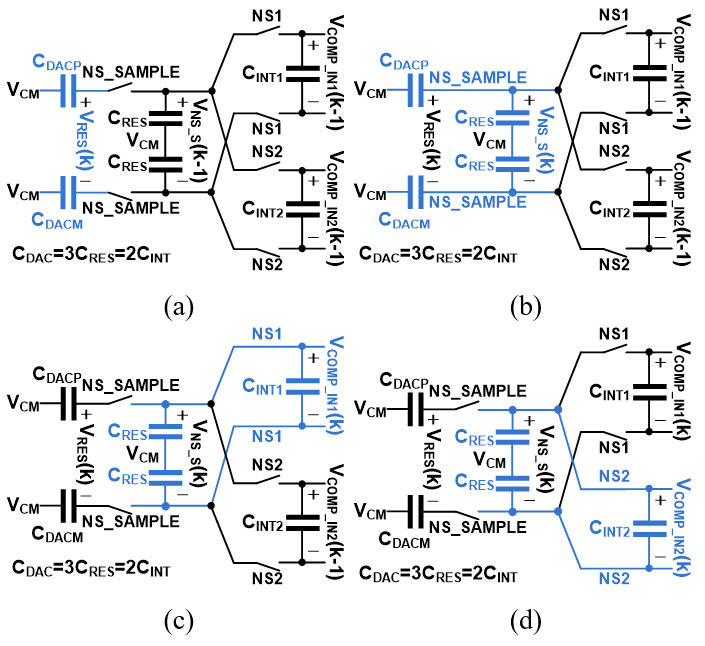### 3. Three-input Comparator with Voltage Gain Calibration

In the behavioral simulation of Section II, the voltage gains of the comparator for V$_{\mathrm{INT1}}$ and V$_{\mathrm{INT2}}$, k$_{1}$ and k$_{2}$, were set to 4 and 16, respectively. Variations of k$_{1}$ and k$_{2}$ values cause degradation of the performance of the NS SAR ADC. Fig. 8 shows the behavioral simulation results for the performance of the NS SAR ADC according to the voltage gain of the comparator with three inputs. When k$_{2}$ is 16, the NS SAR ADC has a stable ENOB when k$_{1}$ is set to a value greater than 3 and less than 5. When k$_{1}$ is set to 4, k$_{2}$ is required to have values between 10 and 22. Thus, in this work, the voltage gain for the three-input comparator is calibrated for optimum performance of the NS SAR ADC.

##### Fig. 8. Behavioral simulation results of NS SAR ADC according to k$_{1}$ and k$_{2}$: (a) when k$_{2}$ = 16; (b) when k$_{1}$ = 4.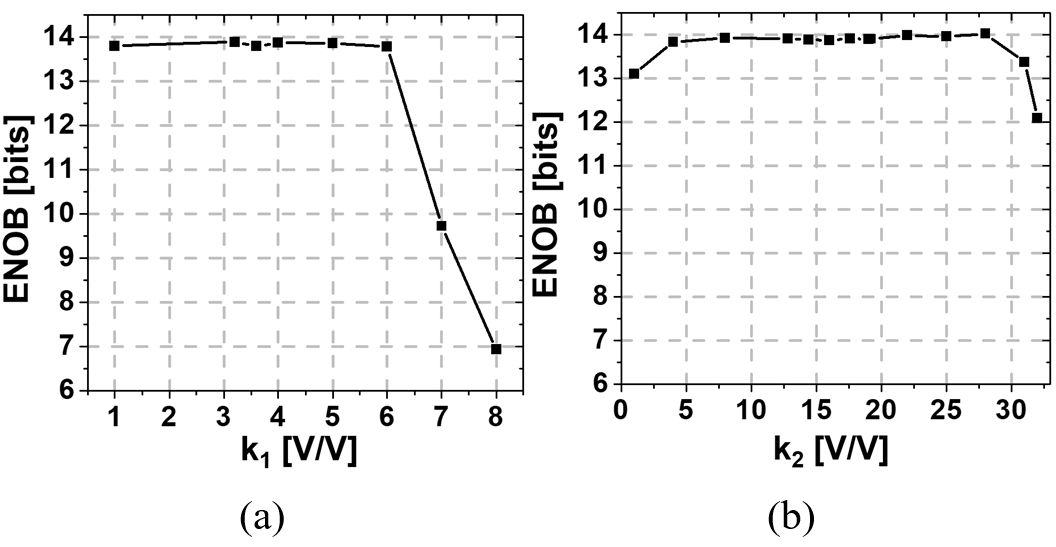Through the behavioral simulation for the maximum performance of the proposed NS SAR ADC, the voltage gain of the three-input comparator for the three input signals (the result of the 10-bit DAC, the output of the first integrator and the output of the second integrator) is determined to be 1:4:16. Fig. 9 describes the conceptual operation of the proposed voltage gain calibration for the three-input comparator. The voltage gain for the output of the first integrator in the three-input comparator should be four times that for the output signal of the 10-bit DAC. As the first step, the 10-bit DAC generates V$_{\mathrm{RES}}$ through the fixed digital codes CP[9:0] and CM[9:0]. After that, V$_{\mathrm{RES}}$ is converted to a voltage of V$_{\mathrm{RES}}$/4 through the sampling process to C$_{\mathrm{RES}}$ and charge sharing between C$_{\mathrm{RES}}$ and C$_{\mathrm{INT1}}$. And the converted differential V$_{\mathrm{RES}}$ /4 is supplied to INTP1 and INTM1 of the three-input comparator, as shown in Fig. 9(a). In the second step, the 10-bit DAC is supplied with a digital code opposite to that of the first step as shown in Fig. 9(b). Then, it generates a V$_{\mathrm{RES}}$ for INP and INM with opposite polarity to the value of V$_{\mathrm{RES}}$/4 generated for INTP1 and INTM1. In this condition, the voltage gain of the three-input comparator is adjusted so that the values of INP-INM'' and INP1-INM1'' are equal, and as a result, the voltage gain of the input terminal for the output of the first integrator is calibrated to 4. In this case, both the nodes INP2 and INM2 remain V$_{\mathrm{CM}}$. The voltage gain calibration of the three-input comparator for the output of the second integrator is almost the same as the method shown in Fig. 9, but instead of V$_{\mathrm{RES}}$/4, V$_{\mathrm{RES}}$/16 is supplied to INP2 and INM2. For this, charge sharing is additionally performed using both C$_{\mathrm{INT1}}$ and C$_{\mathrm{INT2}}$ in the process shown in Fig. 9(a).

##### Fig. 9. Proposed voltage gain calibration of 3-input comparator for output of first integrator: (a) generation of V$_{\mathrm{RES}}$/4; (b) voltage gain control.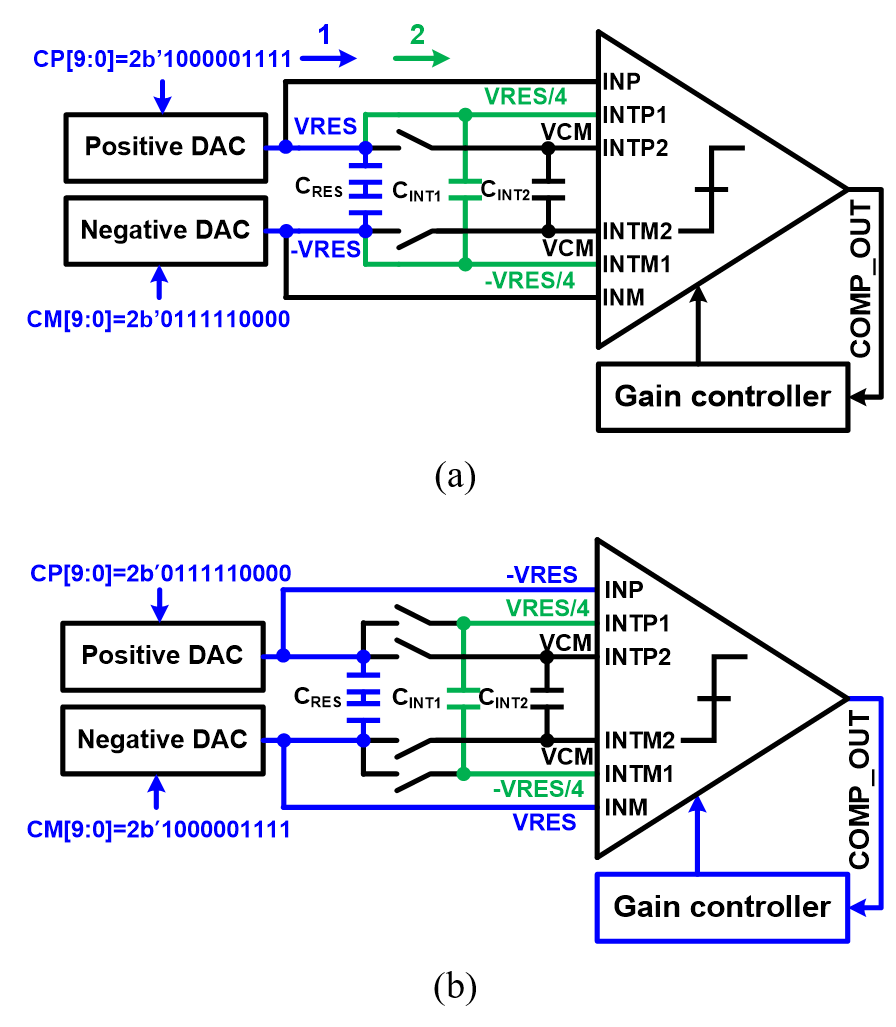Fig. 10 shows the circuit diagram of three-input comparator with voltage gain calibration. When the gains of the input stage are expressed as G1, G2, G3, and G4, the output voltage of the three-input comparator is determined as G4 ${\times}$ (INP ${-}$ INM) + G3 ${\times}$ (G2 ${\times}$ (INTP2 ${-}$ INTM2) + G1 ${\times}$ (INTP1 ${-}$ INTM1)). When the ratios of G4 to G3 and G1 to G2 are 1:2 and 2:8, respectively, the ratio of k$_{1}$ and k$_{2}$ is determined to be 4:16. The proposed voltage gain calibration for the three-input comparator is performed by controlling the ratio of G1 and G2. G1 and G2 are each controlled by changing the width of the input transistors through the control of a 3-bit digital code. Fig. 11 shows the simulated values of k$_{1}$ and k$_{2}$ of the designed three-input comparator including a constant-gm bias circuit according to process, voltage, and temperature (PVT) variations. According to the results of Fig. 11, the calibration ranges of k$_{1}$ and k$_{2}$ were designed to be 1.68 to 6.15 and 7.30 to 22.87, respectively, according to the post-layout simulation results using HSPICE.

##### Fig. 10. Circuit diagram of three-input comparator.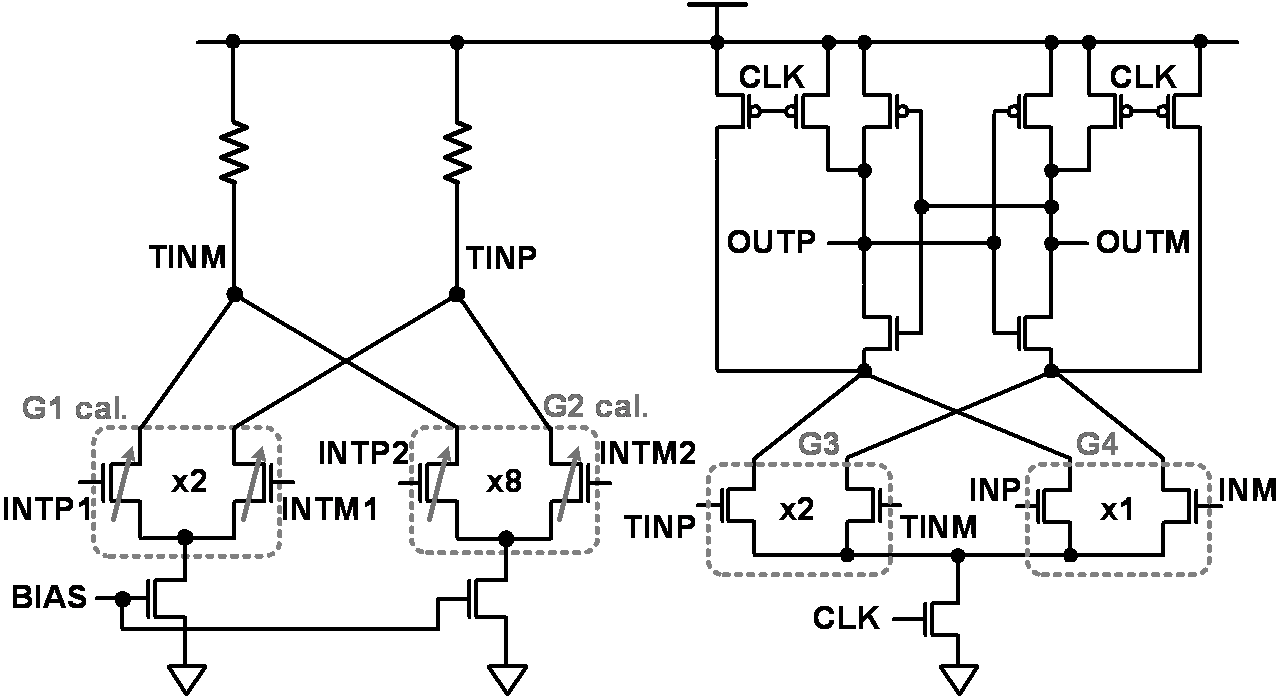##### Fig. 11. Simulated k$_{1}$ and k$_{2}$ of three-input comparator according to PVT variations.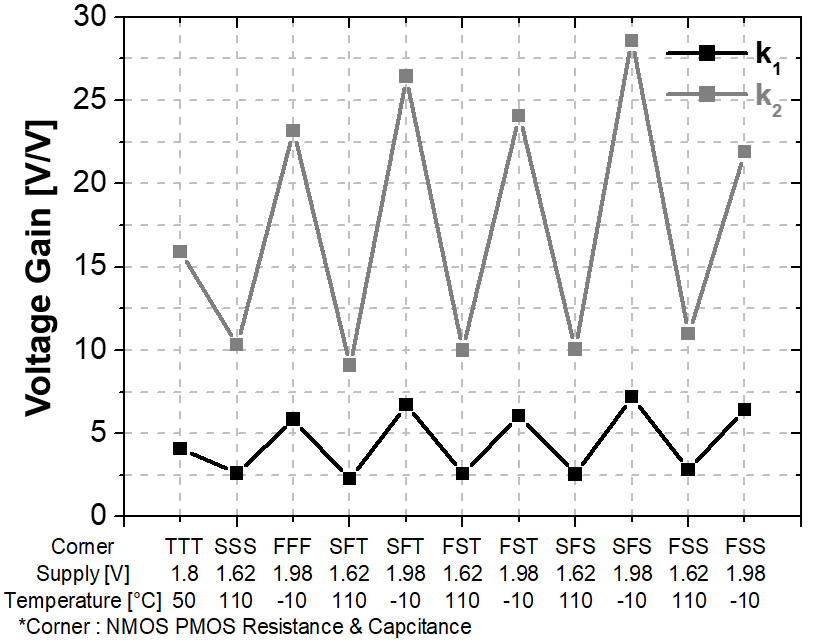## IV. CHIP IMPLEMENTATION AND MEASUREMENT RESULTS

The proposed second-order NS SAR ADC was implemented by using a 180-nm 1-poly 5-metal CMOS process with a supply voltage of 1.8 V. Its active area is 470 ${\mu}$m ${\times}$ 350 ${\mu}$m, as shown in Fig. 12. The CMOS process used in this work supports the minimum value of the capacitor used as the unit capacitor C as 18 fF. Thus, the reduction of the active area of the proposed NS SAR ADC was limited due to the area for the implementation of the capacitors although the use of the C-R DAC and differential integral capacitors. The power consumption of the second-order NS SAR ADC with an input voltage range of full rail-to-rail is 248 ${\mu}$W when it operates at a sampling rate of 1 MHz and has an OSR of 8.

Fig. 13 show the measured static performances of the 10-bit SAR ADC when the implemented second-order NS SAR operates without noise shaping. The differential nonlinearity (DNL) and integral nonlinearity (INL) are +0.19/${-}$0.20 LSBs and +0.26/${-}$0.24 LSBs, respectively, and both values are within +/${-}$0.5 LSBs. The spikes in the measured DNL and INL are considered to be caused by mismatch between capacitors in the C-R DAC. Fig. 14 shows the measured dynamic performances of the 10-bit SAR ADC through the FFT of the results of the second-order NS SAR. The measured signal-to-noise and distortion ratio (SNDR) and ENOB are 58.82 dB and 9.48 bits, respectively, when the 10-bit SAR ADC operates at a sampling rate of 1 MHz for a differential analog input signal with low frequency of 16.259 kHz.

##### Fig. 12. Chip photograph of implemented second-order NS SAR ADC.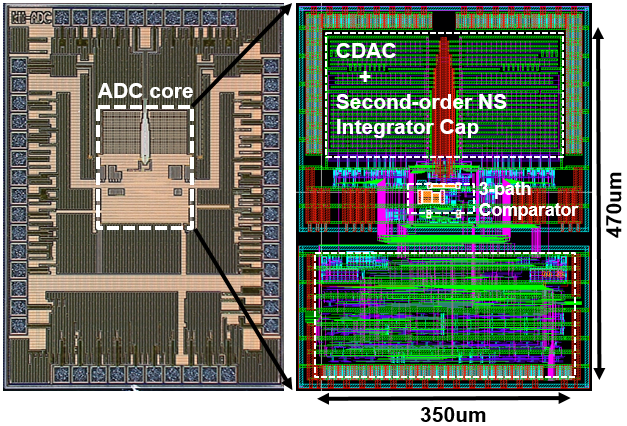##### Fig. 13. Measured static performances of 10-bit SAR ADC: (a) DNL; (b) INL.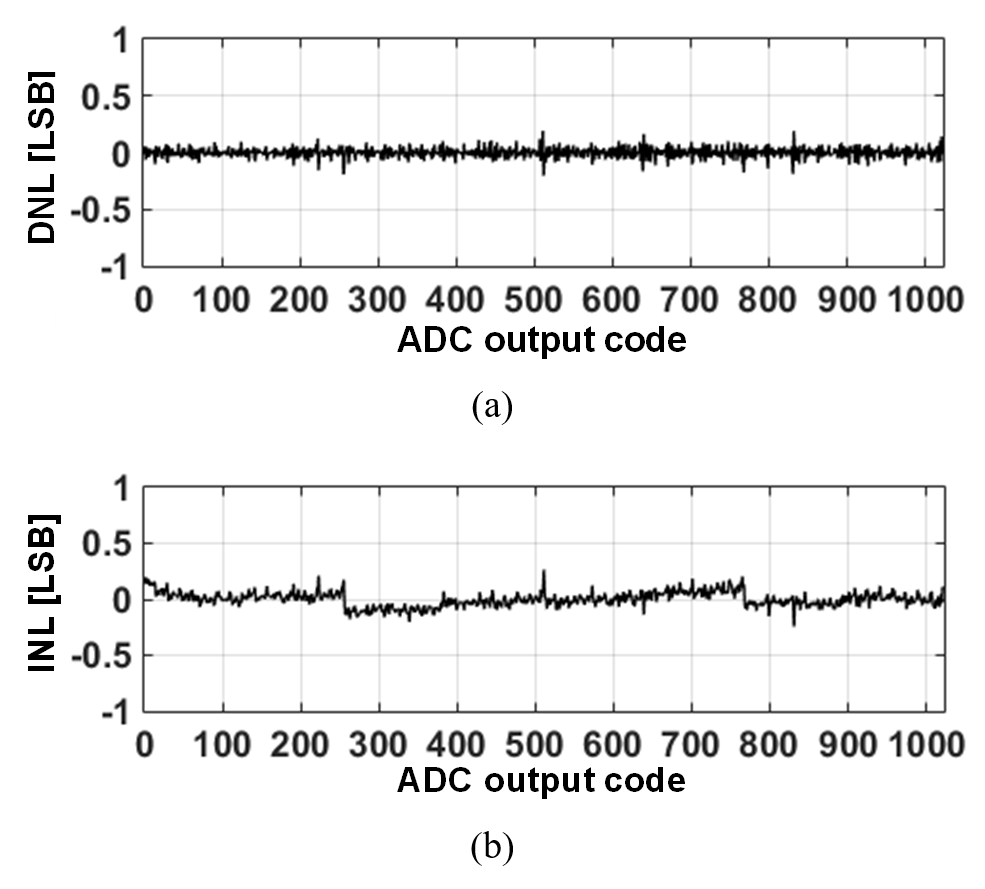##### Fig. 14. Measured dynamic performances of 10-bit SAR ADC.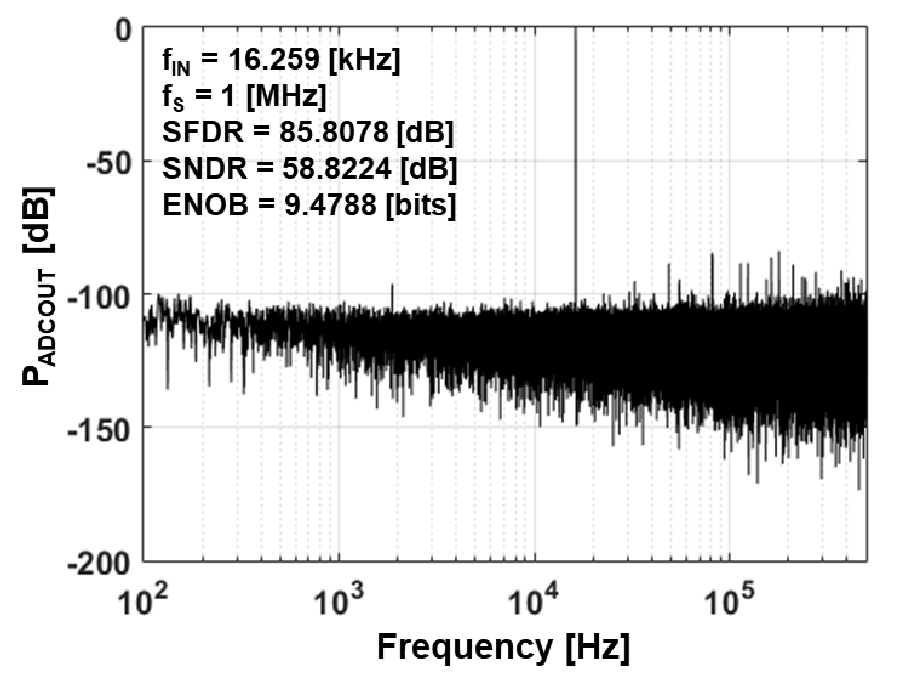Fig. 15 shows the measured dynamic performances of the implemented second-order NS SAR, including the effect of the proposed voltage gain calibration for the comparator with three inputs. The proposed second-order noise shaping reduced the noise floor by about 20 dB in the low-frequency region compared to the noise floor of the 10-bit SAR ADC shown in Fig. 14. In addition, it moved the quantization noise from the low-frequency region to the high-frequency region. When the implemented second-order NS SAR ADC with an OSR of 8 operates in the initial state without the proposed voltage gain calibration for the three-input comparator, the measured SNDR and ENOB are 78.82 dB and 12.80 bits, respectively, as shown in Fig. 15(a). Fig. 15(b) shows the improved dynamic performance by applying the proposed voltage gain calibration for the three-input comparator. The SNDR and ENOB are measured to be 80.18 dB and 13.03 bits.

##### Fig. 15. Measured dynamic performances of second-order NS SAR ADC: (a) w/o calibration; (b) w/ calibration.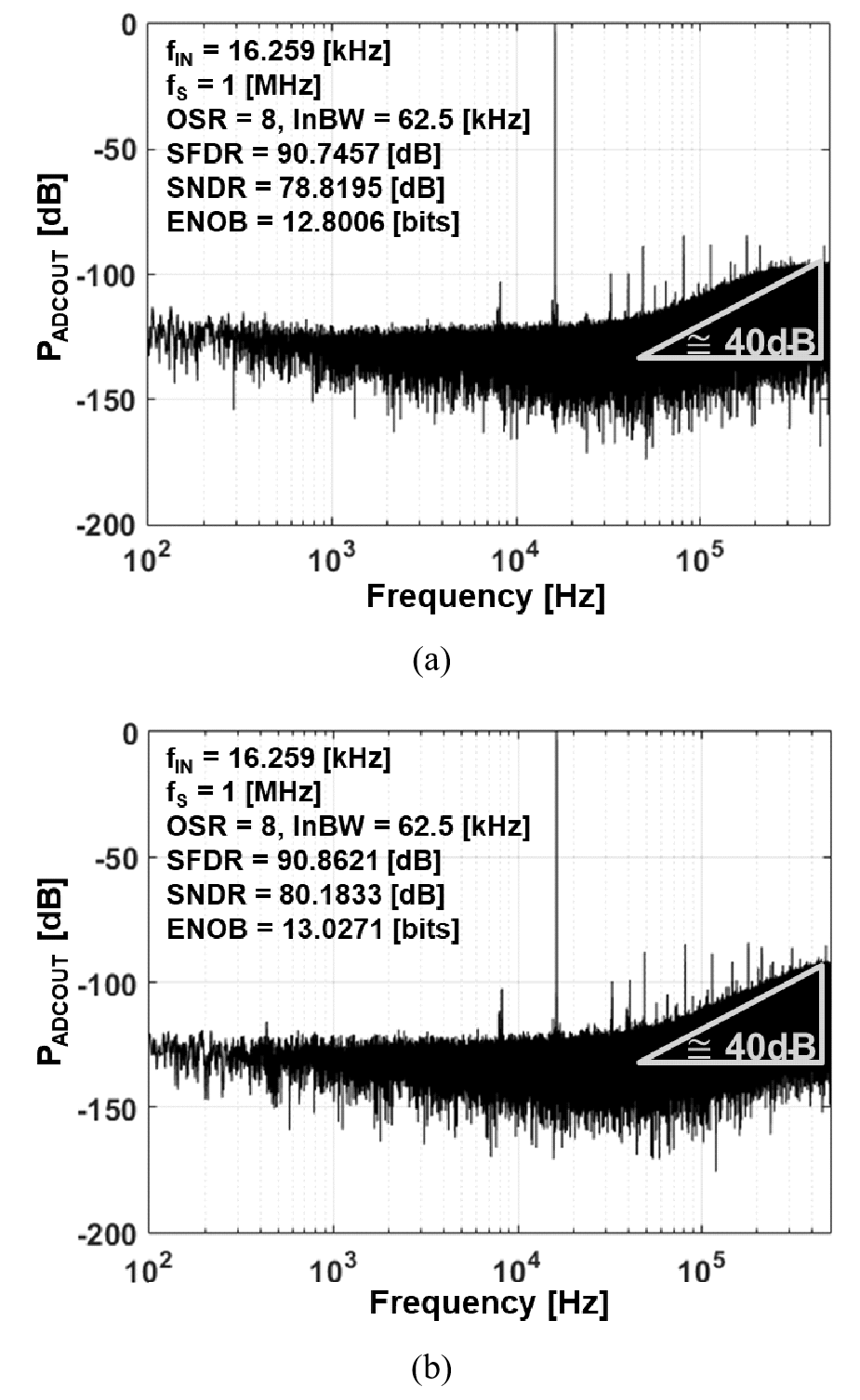Fig. 16 shows the measured SNDR of the proposed second-order NS SAR as the voltage gains of the three-input comparator, k$_{1}$ and k$_{2}$, are varied within the designed range. The implemented second-order NS ADC has optimal SNDR characteristics when the proposed voltage gain calibration is applied rather than when the voltage gains of the comparator are controlled by external control (Case1, 2, 4, and 5). Fig. 17 shows the measured SNDR of the proposed second-order NS SAR ADC according to the amplitude of the analog input signal with a frequency of 16.259 kHz.

##### Fig. 16. Measured SNDR of second-order NS SAR ADC according to voltage gains of comparator (k$_{1}$, and k$_{2}$).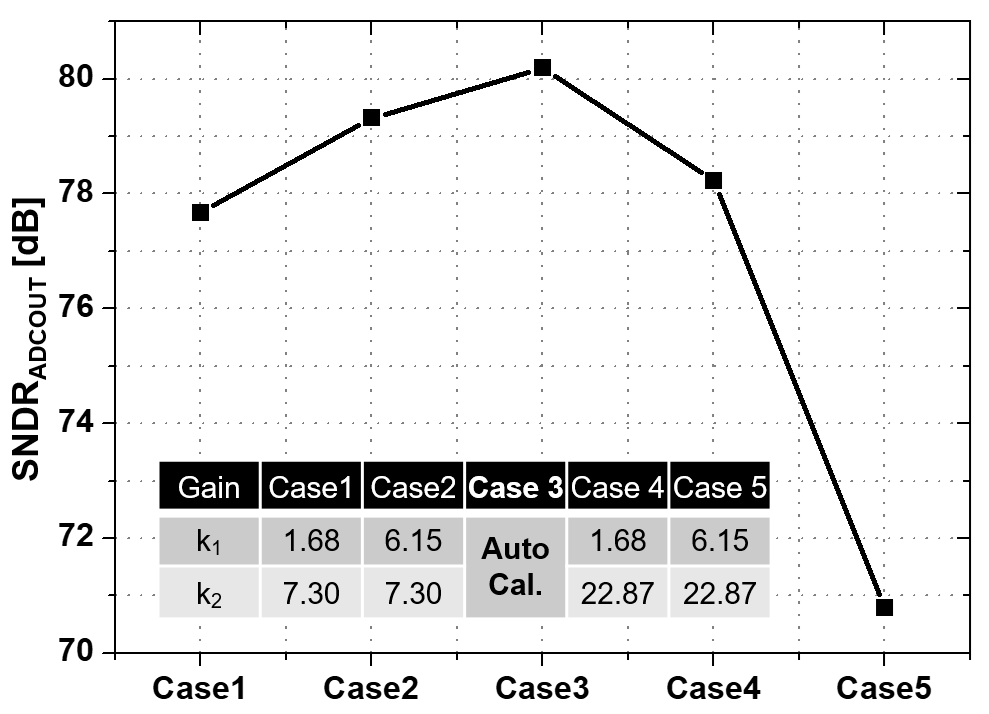##### Fig. 17. Measured SNDR of second-order NS SAR ADC according to amplitude of input signal.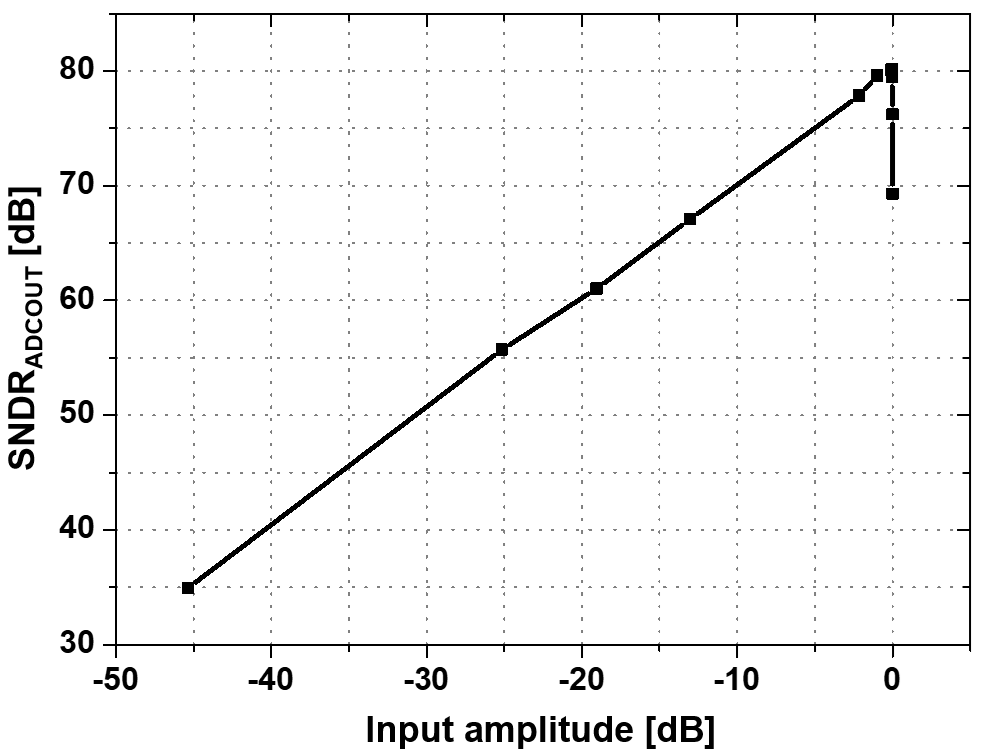Table 2 shows the performance comparison of NS SAR ADCs. The proposed second-order NS ADC has an ENOB of 13 bits or more while it has an OSR of 8.

##### Table 2. Performance comparison of NS SAR ADCs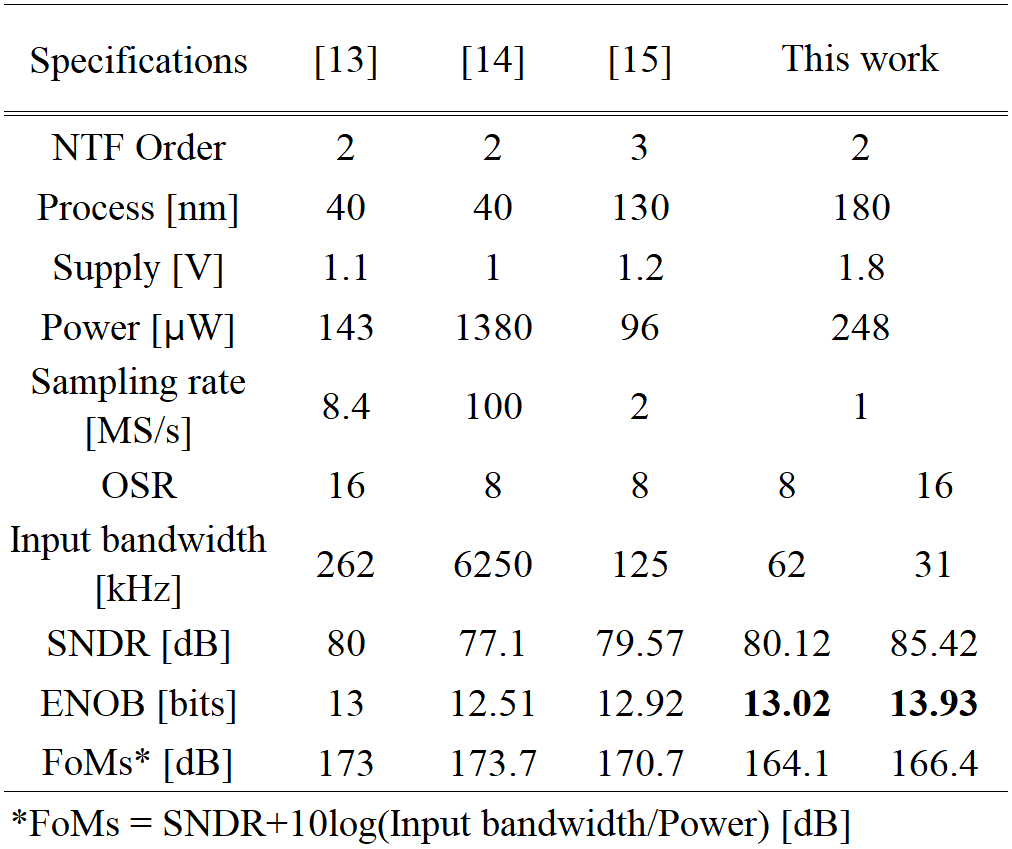## V. CONCLUSIONS

The proposed second-order NS SAR ADC with the passive integrators was designed by using a 180-nm CMOS process with a supply voltage of 1.8 V. The C-R DAC and two differential integrals were used to reduce the active area. Furthermore, the proposed voltage gain calibration for the three-input comparator maximized the dynamic performance of the second NS SAR ADC. The second noise shaping applied to the 10-bit SAR ADC improved a SNDR of 21.36 dB, equivalent to an ENOB of 3.55. Thus, the proposed second-order NS SAR ADC had a SNDR of 80.18 dB and an ENOB of 13.03 bits for an analog input signal with a voltage range of full rail-to-rail and with a frequency of 16.259 kHz while it has an OSR of 8 and a sampling rate of 1 MHz. Its area and power consumption were 0.165 mm$^{2}$ and 248 ${\mu}$W, respectively.

## ACKNOWLEDGMENTS

This work was supported by the Basic Science Research Program (2020R1I1A3071634) and Priority Research Centers Program (2018R1A6A1A03024003) through the NRF funded by the Ministry of Education, and the Grand Information Technology Research Center Program (IITP-2022-2020-0-01612) through the IITP funded by the MSIT, Korea. The EDA tool was supported by the IC Design Education Center, Korea.

## References

1
Liu C.-C., Huang M-C., Feb. 2017, A 0.46mW 5MHz-BW 79.7dB-SNDR Noise-Shaping SAR ADC with Dynamic-Amplifier-Based FIR-IIR Filter, IEEE International Solid-State Circuits Conference, pp. 466-4672
Li S., Qiao B., Gandara M., Pan D. Z., Sun N., Dec. 2018, A 13-ENOB second-order noise-shaping SAR ADC realizing optimized NTF zeros using the error-feedback structure, IEEE Journal of Solid-State Circuits, Vol. 53, No. 12, pp. 3484-34963
Karmakar S., Gònen B., Sebastiano F., Van Veldhoven R., Makinwa K. A. A., Dec. 2018, A 280 μW dynamic-zoom ADC with 120 dB DR and 118 dB SNDR in 1 kHz BW, IEEE Journal of Solid-State Circuits, Vol. 53, No. 12, pp. 3497-35074
Shu Y.-S., Kuo L.-T., Lo T.-Y., Dec. 2016, An oversampling SAR ADC with DAC mismatch error shaping achieving 105 dB SFDR and 101 dB SNDR over 1 kHz BW in 55 nm CMOS, IEEE Journal of Solid-State Circuits, Vol. 51, No. 12, pp. 2928-29405
Miyahara M., Matsuzawa A., Apr. 2017, An 84 dB dynamic range 62.5-625 kHz bandwidth clock-scalable noise-shaping SAR ADC with open-loop integrator using dynamic amplifier, IEEE Custom Integrated Circuits Conference, pp. 1-46
Akbari M., Honarparvar M., Savaria Y., Sawan M., Oct. 2020, OTA-free MASH 2-2 noise shaping SAR ADC: System and design considera-tions, 2020 IEEE International Symposium on Circuits and Systems, pp. 1-57
Zhang Q., Ning N., Li J., Yu Q., Wu K., Zhang Z., Jan. 2020, A Second-Order Noise-Shaping SAR ADC Using Two Passive Integrators Separated by the Comparator, IEEE Transactions on Very Large Scale Integration Systems, Vol. 29, No. 1, pp. 227-2318
Lin Y.-Z., Lin C.-Y., Tsou S.-C., Tsai C.-H., Lu C.-H., Feb. 2019, A 40MHz-BW 320MS/s Passive Noise-Shaping SAR ADC with Passive Signal-Residue Summation in 14nm FinFET, IEEE International Solid-State Circuits Conference, pp. 330-3329
Shiet L., Zhang Y., Wang Y., Kareppagoudr M., Sadollahi M., Temes G. C., Aug. 2018, A 13b-ENOB noise shaping SAR ADC with a two-capacitor DAC, International Midwest Symposium on Circuits and Systems, pp. 153-15610
Jung H., Jeon N., Jang Y.-C., Oct. 2021, Second-order Noise Shaping SAR ADC using 3-input Comparator with Voltage Gain Calibration, International SoC Design Conference, pp. 123-12411
Jung H., Dec. 2020, Study on Second-order Noise Shaping SAR ADC, Master Degee, Kumoh National Institute od Technology12
Park J., Nagaraj K., Ash M., Kumar A., Apr. 2017, A 12-/14-bit, 4/2MSPS, 0.085 mm2 SAR ADC in 65nm using novel residue boosting, IEEE Custom Integrated Circuits Conference, pp. 2152-363013
Guo W., Zhuang H., Sun N., Jun. 2017, A 13b-ENOB 173dB-FoM 2 nd-order NS SAR ADC with passive integrators, IEEE Symposium on VLSI Circuits, pp. c236-C23714
Hsu C.-K., Tang X., Liu J., Xu R., Zhao W., Mukherjee A., Andeen T. R., Sun N., Mar. 2021, A 77.1-dB-SNDR 6.25-MHz-BW pipeline SAR ADC with enhanced interstage gain error shaping and quantization noise shaping, IEEE Journal of Solid-State Circuits, Vol. 56, No. 3, pp. 739-74015
Zhang Q., Li J., Zhang Z., Wu K., Ning N., Yu Q., Ari. 2021, A 13b-ENOB third-order noise-shaping SAR ADC using a hybrid error-control structure, IEEE Custom Integrated Circuits Conference, pp. 1-2Hoyong Jung was born in Busan, South Korea, in 1994. He received the B.S. and M.S. degrees from the Department of Electronic Engi-neering, Kumoh National Institute of Technology, Gumi, South Korea, in 2019 and 2021, respectively, where he is currently pursuing the Ph.D. degree. His current research interests include the design of data converters.

Neung-in Jeon was born in Ulsan, South Korea, in 1996. He received the B.S. degrees in the department of electronic engineering from Silla University, Busan, Korea, in 2021. He is currently pursuing the M.S. degrees in the Department of Electronic Engineering, Kumoh National Institute of Technology, Gumi, South Korea. His current research interests include the design of data converters.

Jimin Cheon received the B.S., M.S., and Ph.D. degrees in electrical and electronic engineering from Yonsei University, Seoul, South Korea, in 2003, 2005, and 2010, respectively. From 2010 to 2012, he was a Senior Engineer in the Image Development Team of System LSI Division, Samsung Electronics, Yongin, South Korea, working on the design of APS-C CMOS image sensors for mirrorless and DSLR cameras. From 2012 to 2013, he was a Manager in the Semiconductor Tech. Laboratory of Fusion Technology Research and Development Center, SK Telecom, Seongnam, South Korea, working on the design of automotive CMOS image sensors with SK hynix. In 2013, he joined the School of Electronic Engineering, Kumoh National Institute of Technology, Gumi, Korea, as a Faculty Member. His current research area is the design of CMOS image sensors, low-power data converters, high performance sensors, and interface circuits.

Young-Chan Jang received the B.S. degree in the department of elec¬tronic engineering from Kyungpook National University, Daegu, Korea, in 1999 and the M.S. and Ph.D. degrees in electronic engineering from Pohang University of Science and Technology (POSTECH), Pohang, Korea, in 2001 and 2005, respectively. From 2005 to 2009, he was a Senior Engineer in the Memory Division, Samsung Electronics, Hwasung, Korea, working on high-speed interface circuit design and next-generation DRAM. In 2009, he joined the School of Electronic Engineering, Kumoh National Institute of Technology, Gumi, Korea, as a Faculty Member, where he is currently Professor. His current research area is high-performance mixed-mode circuit design for VLSI systems such as high-performance signaling, clock generation, and analog-to-digital conversion.